Feature Columns

This document details feature columns. Think of feature columns as the intermediaries between raw data and Estimators. Feature columns are very rich, enabling you to transform a diverse range of raw data into formats that Estimators can use, allowing easy experimentation.

In Premade Estimators, we used the premade Estimator, `tf.estimator.DNNClassifier` to train a model to predict different types of Iris flowers from four input features. That example created only numerical feature columns (of type `tf.feature_column.numeric_column`). Although numerical feature columns model the lengths of petals and sepals effectively, real world data sets contain all kinds of features, many of which are non-numerical.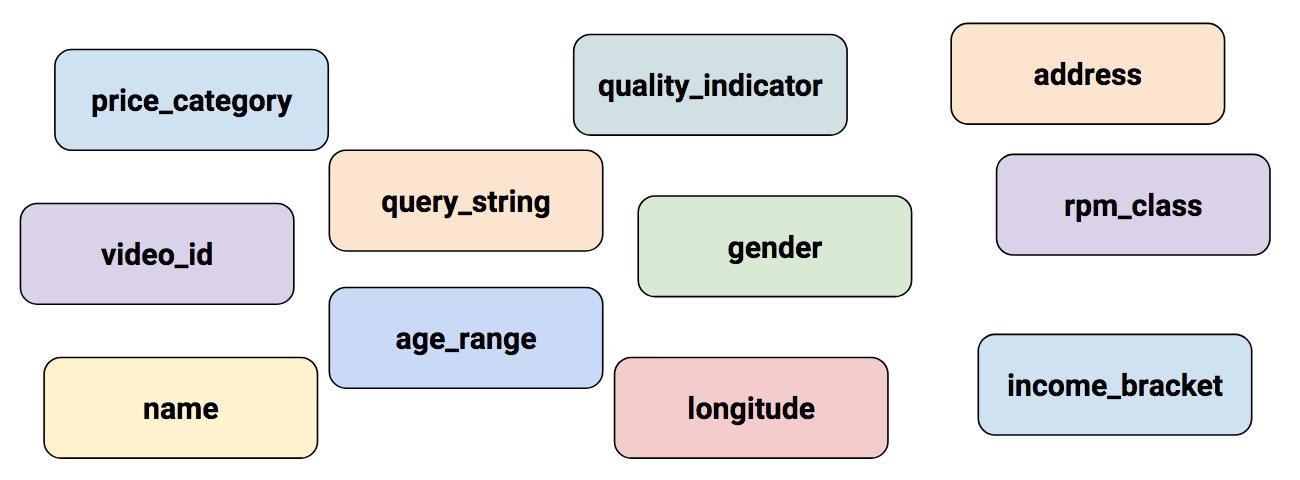Some real-world features (such as, longitude) are numerical, but many are not.

Input to a Deep Neural Network

What kind of data can a deep neural network operate on? The answer is, of course, numbers (for example, `tf.float32`). After all, every neuron in a neural network performs multiplication and addition operations on weights and input data. Real-life input data, however, often contains non-numerical (categorical) data. For example, consider a `product_class` feature that can contain the following three non-numerical values:

• `kitchenware`
• `electronics`
• `sports`

ML models generally represent categorical values as simple vectors in which a 1 represents the presence of a value and a 0 represents the absence of a value. For example, when `product_class` is set to `sports`, an ML model would usually represent `product_class` as `[0, 0, 1]`, meaning:

• `0`: `kitchenware` is absent
• `0`: `electronics` is absent
• `1`: `sports` is present

So, although raw data can be numerical or categorical, an ML model represents all features as numbers.

Feature Columns

As the following figure suggests, you specify the input to a model through the `feature_columns` argument of an Estimator (`DNNClassifier` for Iris). Feature Columns bridge input data (as returned by `input_fn`) with your model.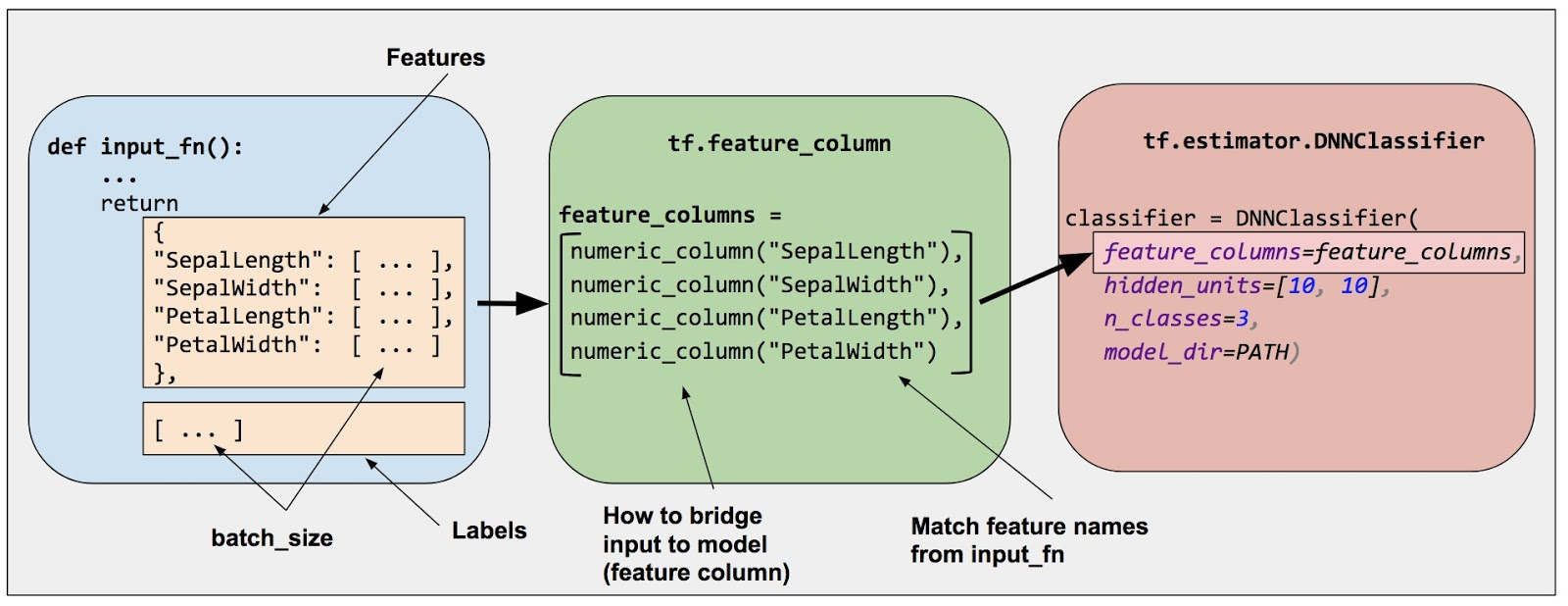Feature columns bridge raw data with the data your model needs.

To create feature columns, call functions from the `tf.feature_column` module. This document explains nine of the functions in that module. As the following figure shows, all nine functions return either a Categorical-Column or a Dense-Column object, except `bucketized_column`, which inherits from both classes: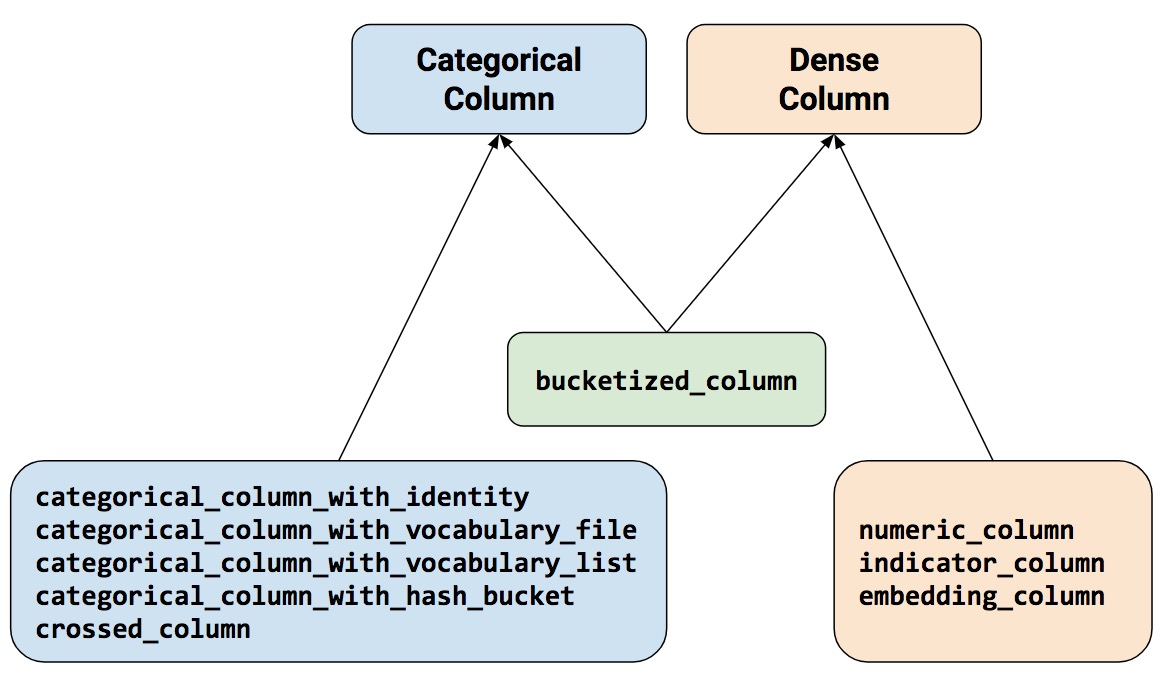Feature column methods fall into two main categories and one hybrid category.

Let's look at these functions in more detail.

Numeric column

The Iris classifier calls the `tf.feature_column.numeric_column` function for all input features:

• `SepalLength`
• `SepalWidth`
• `PetalLength`
• `PetalWidth`

Although `tf.numeric_column` provides optional arguments, calling `tf.numeric_column` without any arguments, as follows, is a fine way to specify a numerical value with the default data type (`tf.float32`) as input to your model:

``````# Defaults to a tf.float32 scalar.
numeric_feature_column = tf.feature_column.numeric_column(key="SepalLength")
``````

To specify a non-default numerical data type, use the `dtype` argument. For example:

``````# Represent a tf.float64 scalar.
numeric_feature_column = tf.feature_column.numeric_column(key="SepalLength",
dtype=tf.float64)
``````

By default, a numeric column creates a single value (scalar). Use the shape argument to specify another shape. For example:

``````# Represent a 10-element vector in which each cell contains a tf.float32.
vector_feature_column = tf.feature_column.numeric_column(key="Bowling",
shape=10)

# Represent a 10x5 matrix in which each cell contains a tf.float32.
matrix_feature_column = tf.feature_column.numeric_column(key="MyMatrix",
shape=[10,5])
``````

Bucketized column

Often, you don't want to feed a number directly into the model, but instead split its value into different categories based on numerical ranges. To do so, create a `tf.feature_column.bucketized_column`. For example, consider raw data that represents the year a house was built. Instead of representing that year as a scalar numeric column, we could split the year into the following four buckets: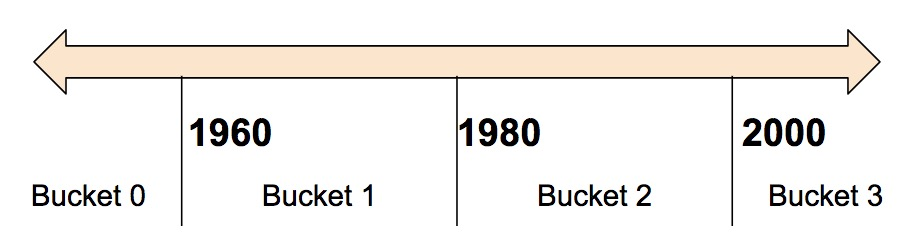Dividing year data into four buckets.

The model will represent the buckets as follows:

Date Range Represented as...
< 1960 [1, 0, 0, 0]
>= 1960 but < 1980 [0, 1, 0, 0]
>= 1980 but < 2000 [0, 0, 1, 0]
>= 2000 [0, 0, 0, 1]

Why would you want to split a number—a perfectly valid input to your model—into a categorical value? Well, notice that the categorization splits a single input number into a four-element vector. Therefore, the model now can learn four individual weights rather than just one; four weights creates a richer model than one weight. More importantly, bucketizing enables the model to clearly distinguish between different year categories since only one of the elements is set (1) and the other three elements are cleared (0). For example, when we just use a single number (a year) as input, a linear model can only learn a linear relationship. So, bucketing provides the model with additional flexibility that the model can use to learn.

The following code demonstrates how to create a bucketized feature:

``````# First, convert the raw input to a numeric column.
numeric_feature_column = tf.feature_column.numeric_column("Year")

# Then, bucketize the numeric column on the years 1960, 1980, and 2000.
bucketized_feature_column = tf.feature_column.bucketized_column(
source_column = numeric_feature_column,
boundaries = [1960, 1980, 2000])
``````

Note that specifying a three-element boundaries vector creates a four-element bucketized vector.

Categorical identity column

Categorical identity columns can be seen as a special case of bucketized columns. In traditional bucketized columns, each bucket represents a range of values (for example, from 1960 to 1979). In a categorical identity column, each bucket represents a single, unique integer. For example, let's say you want to represent the integer range `[0, 4)`. That is, you want to represent the integers 0, 1, 2, or 3. In this case, the categorical identity mapping looks like this: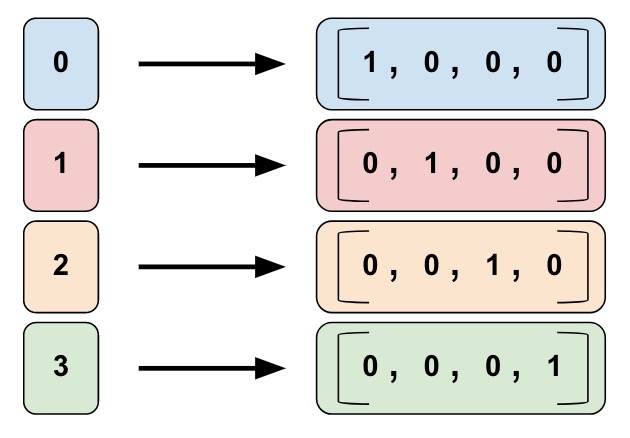A categorical identity column mapping. Note that this is a one-hot encoding, not a binary numerical encoding.

As with bucketized columns, a model can learn a separate weight for each class in a categorical identity column. For example, instead of using a string to represent the `product_class`, let's represent each class with a unique integer value. That is:

• `0="kitchenware"`
• `1="electronics"`
• `2="sport"`

Call `tf.feature_column.categorical_column_with_identity` to implement a categorical identity column. For example:

``````# Create categorical output for an integer feature named "my_feature_b",
# The values of my_feature_b must be >= 0 and < num_buckets
identity_feature_column = tf.feature_column.categorical_column_with_identity(
key='my_feature_b',
num_buckets=4) # Values [0, 4)

# In order for the preceding call to work, the input_fn() must return
# a dictionary containing 'my_feature_b' as a key. Furthermore, the values
# assigned to 'my_feature_b' must belong to the set [0, 4).
def input_fn():
...
return ({ 'my_feature_a':[7, 9, 5, 2], 'my_feature_b':[3, 1, 2, 2] },
[Label_values])
``````

Categorical vocabulary column

We cannot input strings directly to a model. Instead, we must first map strings to numeric or categorical values. Categorical vocabulary columns provide a good way to represent strings as a one-hot vector. For example: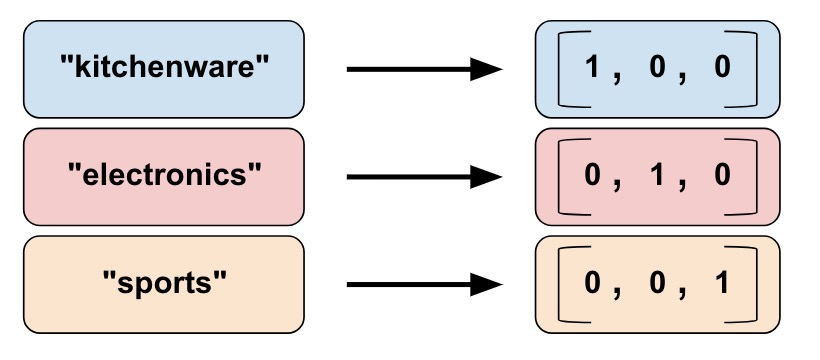Mapping string values to vocabulary columns.

As you can see, categorical vocabulary columns are kind of an enum version of categorical identity columns. TensorFlow provides two different functions to create categorical vocabulary columns:

`categorical_column_with_vocabulary_list` maps each string to an integer based on an explicit vocabulary list. For example:

``````# Given input "feature_name_from_input_fn" which is a string,
# create a categorical feature by mapping the input to one of
# the elements in the vocabulary list.
vocabulary_feature_column =
tf.feature_column.categorical_column_with_vocabulary_list(
key=feature_name_from_input_fn,
vocabulary_list=["kitchenware", "electronics", "sports"])
``````

The preceding function is pretty straightforward, but it has a significant drawback. Namely, there's way too much typing when the vocabulary list is long. For these cases, call `tf.feature_column.categorical_column_with_vocabulary_file` instead, which lets you place the vocabulary words in a separate file. For example:

``````
# Given input "feature_name_from_input_fn" which is a string,
# create a categorical feature to our model by mapping the input to one of
# the elements in the vocabulary file
vocabulary_feature_column =
tf.feature_column.categorical_column_with_vocabulary_file(
key=feature_name_from_input_fn,
vocabulary_file="product_class.txt",
vocabulary_size=3)
``````

`product_class.txt` should contain one line for each vocabulary element. In our case:

```kitchenware
electronics
sports
```

Hashed Column

So far, we've worked with a naively small number of categories. For example, our product_class example has only 3 categories. Often though, the number of categories can be so big that it's not possible to have individual categories for each vocabulary word or integer because that would consume too much memory. For these cases, we can instead turn the question around and ask, "How many categories am I willing to have for my input?" In fact, the `tf.feature_column.categorical_column_with_hash_bucket` function enables you to specify the number of categories. For this type of feature column the model calculates a hash value of the input, then puts it into one of the `hash_bucket_size` categories using the modulo operator, as in the following pseudocode:

``````# pseudocode
feature_id = hash(raw_feature) % hash_bucket_size
``````

The code to create the `feature_column` might look something like this:

``````hashed_feature_column =
tf.feature_column.categorical_column_with_hash_bucket(
key = "some_feature",
hash_bucket_size = 100) # The number of categories
``````

At this point, you might rightfully think: "This is crazy!" After all, we are forcing the different input values to a smaller set of categories. This means that two probably unrelated inputs will be mapped to the same category, and consequently mean the same thing to the neural network. The following figure illustrates this dilemma, showing that kitchenware and sports both get assigned to category (hash bucket) 12: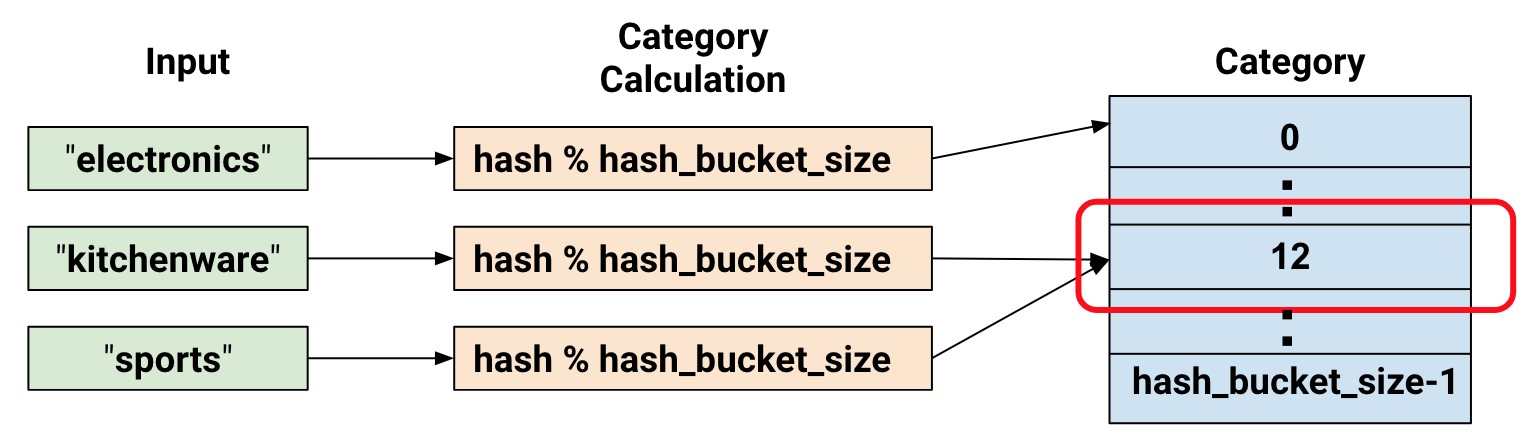Representing data with hash buckets.

As with many counterintuitive phenomena in machine learning, it turns out that hashing often works well in practice. That's because hash categories provide the model with some separation. The model can use additional features to further separate kitchenware from sports.

Crossed column

Combining features into a single feature, better known as feature crosses, enables the model to learn separate weights for each combination of features.

More concretely, suppose we want our model to calculate real estate prices in Atlanta, GA. Real-estate prices within this city vary greatly depending on location. Representing latitude and longitude as separate features isn't very useful in identifying real-estate location dependencies; however, crossing latitude and longitude into a single feature can pinpoint locations. Suppose we represent Atlanta as a grid of 100x100 rectangular sections, identifying each of the 10,000 sections by a feature cross of latitude and longitude. This feature cross enables the model to train on pricing conditions related to each individual section, which is a much stronger signal than latitude and longitude alone.

The following figure shows our plan, with the latitude & longitude values for the corners of the city in red text: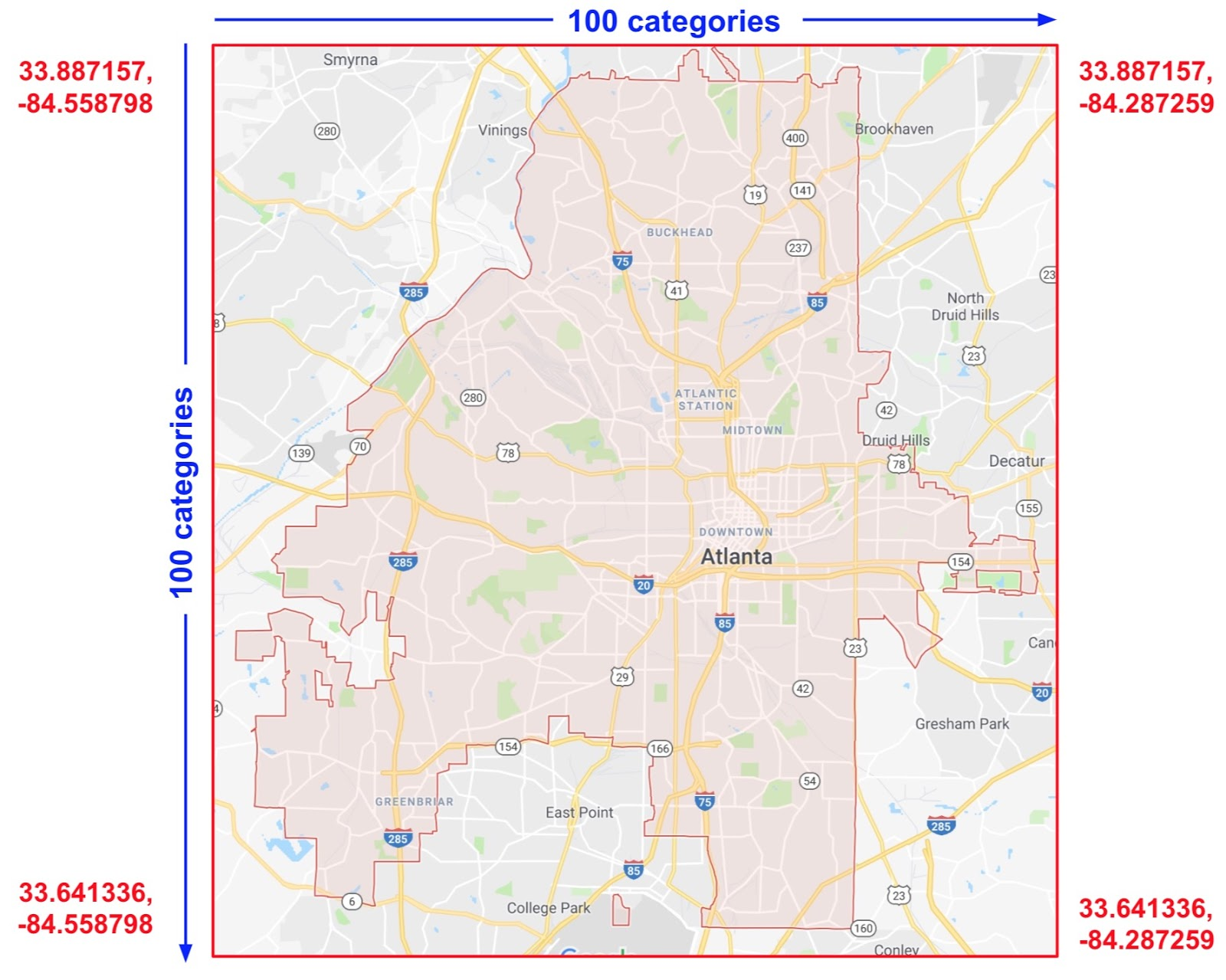Map of Atlanta. Imagine this map divided into 10,000 sections of equal size.

For the solution, we used a combination of the `bucketized_column` we looked at earlier, with the `tf.feature_column.crossed_column` function.

``````def make_dataset(latitude, longitude, labels):
assert latitude.shape == longitude.shape == labels.shape

features = {'latitude': latitude.flatten(),
'longitude': longitude.flatten()}
labels=labels.flatten()

return tf.data.Dataset.from_tensor_slices((features, labels))

# Bucketize the latitude and longitude using the `edges`
latitude_bucket_fc = tf.feature_column.bucketized_column(
tf.feature_column.numeric_column('latitude'),
list(atlanta.latitude.edges))

longitude_bucket_fc = tf.feature_column.bucketized_column(
tf.feature_column.numeric_column('longitude'),
list(atlanta.longitude.edges))

# Cross the bucketized columns, using 5000 hash bins.
crossed_lat_lon_fc = tf.feature_column.crossed_column(
[latitude_bucket_fc, longitude_bucket_fc], 5000)

fc = [
latitude_bucket_fc,
longitude_bucket_fc,
crossed_lat_lon_fc]

# Build and train the Estimator.
est = tf.estimator.LinearRegressor(fc, ...)
``````

You may create a feature cross from either of the following:

• Feature names; that is, names from the `dict` returned from `input_fn`.
• Any categorical column, except `categorical_column_with_hash_bucket` (since `crossed_column` hashes the input).

When the feature columns `latitude_bucket_fc` and `longitude_bucket_fc` are crossed, TensorFlow will create `(latitude_fc, longitude_fc)` pairs for each example. This would produce a full grid of possibilities as follows:

``` (0,0),  (0,1)...  (0,99)
(1,0),  (1,1)...  (1,99)
...     ...       ...
(99,0), (99,1)...(99, 99)
```

Except that a full grid would only be tractable for inputs with limited vocabularies. Instead of building this, potentially huge, table of inputs, the `crossed_column` only builds the number requested by the `hash_bucket_size` argument. The feature column assigns an example to a index by running a hash function on the tuple of inputs, followed by a modulo operation with `hash_bucket_size`.

As discussed earlier, performing the hash and modulo function limits the number of categories, but can cause category collisions; that is, multiple (latitude, longitude) feature crosses will end up in the same hash bucket. In practice though, performing feature crosses still adds significant value to the learning capability of your models.

Somewhat counterintuitively, when creating feature crosses, you typically still should include the original (uncrossed) features in your model (as in the preceding code snippet). The independent latitude and longitude features help the model distinguish between examples where a hash collision has occurred in the crossed feature.

Indicator and embedding columns

Indicator columns and embedding columns never work on features directly, but instead take categorical columns as input.

When using an indicator column, we're telling TensorFlow to do exactly what we've seen in our categorical product_class example. That is, an indicator column treats each category as an element in a one-hot vector, where the matching category has value 1 and the rest have 0s:Representing data in indicator columns.

Here's how you create an indicator column by calling `tf.feature_column.indicator_column`:

``````categorical_column = ... # Create any type of categorical column.

# Represent the categorical column as an indicator column.
indicator_column = tf.feature_column.indicator_column(categorical_column)
``````

Now, suppose instead of having just three possible classes, we have a million. Or maybe a billion. For a number of reasons, as the number of categories grow large, it becomes infeasible to train a neural network using indicator columns.

We can use an embedding column to overcome this limitation. Instead of representing the data as a one-hot vector of many dimensions, an embedding column represents that data as a lower-dimensional, ordinary vector in which each cell can contain any number, not just 0 or 1. By permitting a richer palette of numbers for every cell, an embedding column contains far fewer cells than an indicator column.

Let's look at an example comparing indicator and embedding columns. Suppose our input examples consist of different words from a limited palette of only 81 words. Further suppose that the data set provides the following input words in 4 separate examples:

• `"dog"`
• `"spoon"`
• `"scissors"`
• `"guitar"`

In that case, the following figure illustrates the processing path for embedding columns or indicator columns.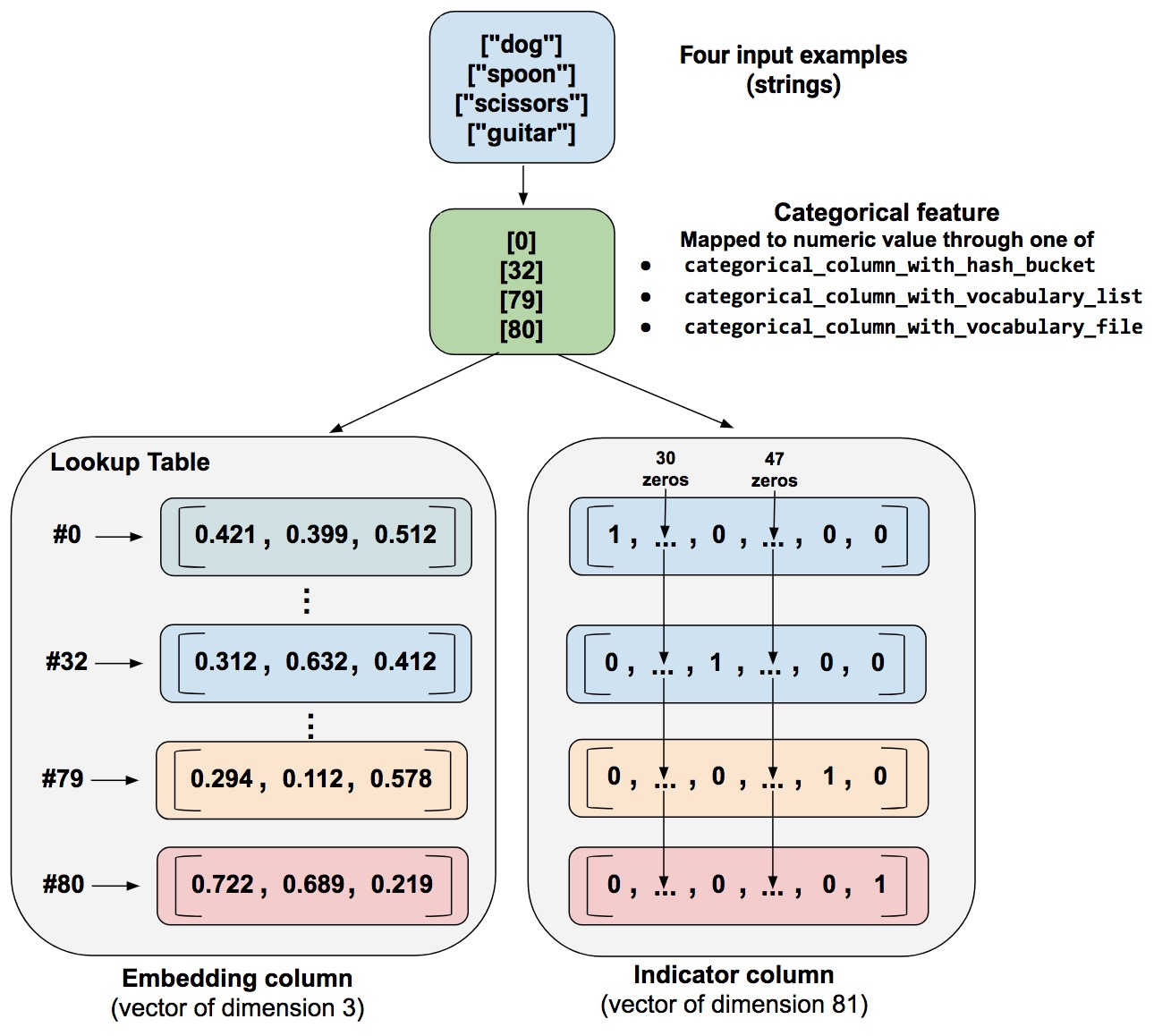An embedding column stores categorical data in a lower-dimensional vector than an indicator column. (We just placed random numbers into the embedding vectors; training determines the actual numbers.)

When an example is processed, one of the `categorical_column_with...` functions maps the example string to a numerical categorical value. For example, a function maps "spoon" to ``. (The 32 comes from our imagination—the actual values depend on the mapping function.) You may then represent these numerical categorical values in either of the following two ways:

• As an indicator column. A function converts each numeric categorical value into an 81-element vector (because our palette consists of 81 words), placing a 1 in the index of the categorical value (0, 32, 79, 80) and a 0 in all the other positions.

• As an embedding column. A function uses the numerical categorical values `(0, 32, 79, 80)` as indices to a lookup table. Each slot in that lookup table contains a 3-element vector.

How do the values in the embeddings vectors magically get assigned? Actually, the assignments happen during training. That is, the model learns the best way to map your input numeric categorical values to the embeddings vector value in order to solve your problem. Embedding columns increase your model's capabilities, since an embeddings vector learns new relationships between categories from the training data.

Why is the embedding vector size 3 in our example? Well, the following "formula" provides a general rule of thumb about the number of embedding dimensions:

``````embedding_dimensions =  number_of_categories**0.25
``````

That is, the embedding vector dimension should be the 4th root of the number of categories. Since our vocabulary size in this example is 81, the recommended number of dimensions is 3:

``````3 =  81**0.25
``````

Call `tf.feature_column.embedding_column` to create an `embedding_column` as suggested by the following snippet:

``````categorical_column = ... # Create any categorical column

# Represent the categorical column as an embedding column.
# This means creating an embedding vector lookup table with one element for each category.
embedding_column = tf.feature_column.embedding_column(
categorical_column=categorical_column,
dimension=embedding_dimensions)
``````

Embeddings is a significant topic within machine learning. This information was just to get you started using them as feature columns.

Passing feature columns to Estimators

As the following list indicates, not all Estimators permit all types of `feature_columns` argument(s):

• `tf.estimator.LinearClassifier` and `tf.estimator.LinearRegressor`: Accept all types of feature column.
• `tf.estimator.DNNClassifier` and `tf.estimator.DNNRegressor`: Only accept dense columns. Other column types must be wrapped in either an `indicator_column` or `embedding_column`.
• `tf.estimator.DNNLinearCombinedClassifier` and `tf.estimator.DNNLinearCombinedRegressor`:
• The `linear_feature_columns` argument accepts any feature column type.
• The `dnn_feature_columns` argument only accepts dense columns.

Other Sources

For more examples on feature columns, view the following: